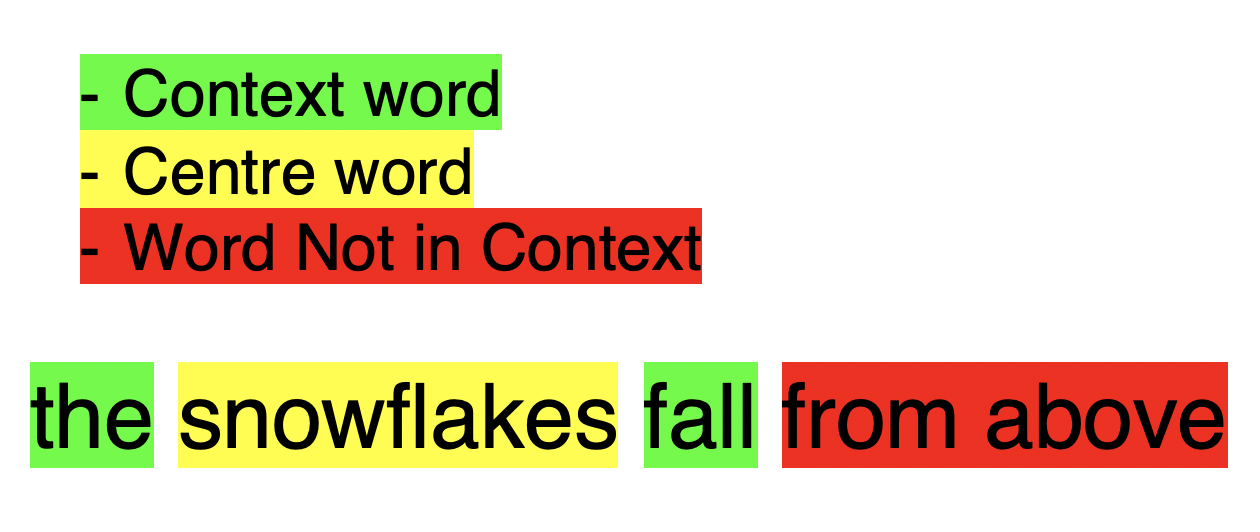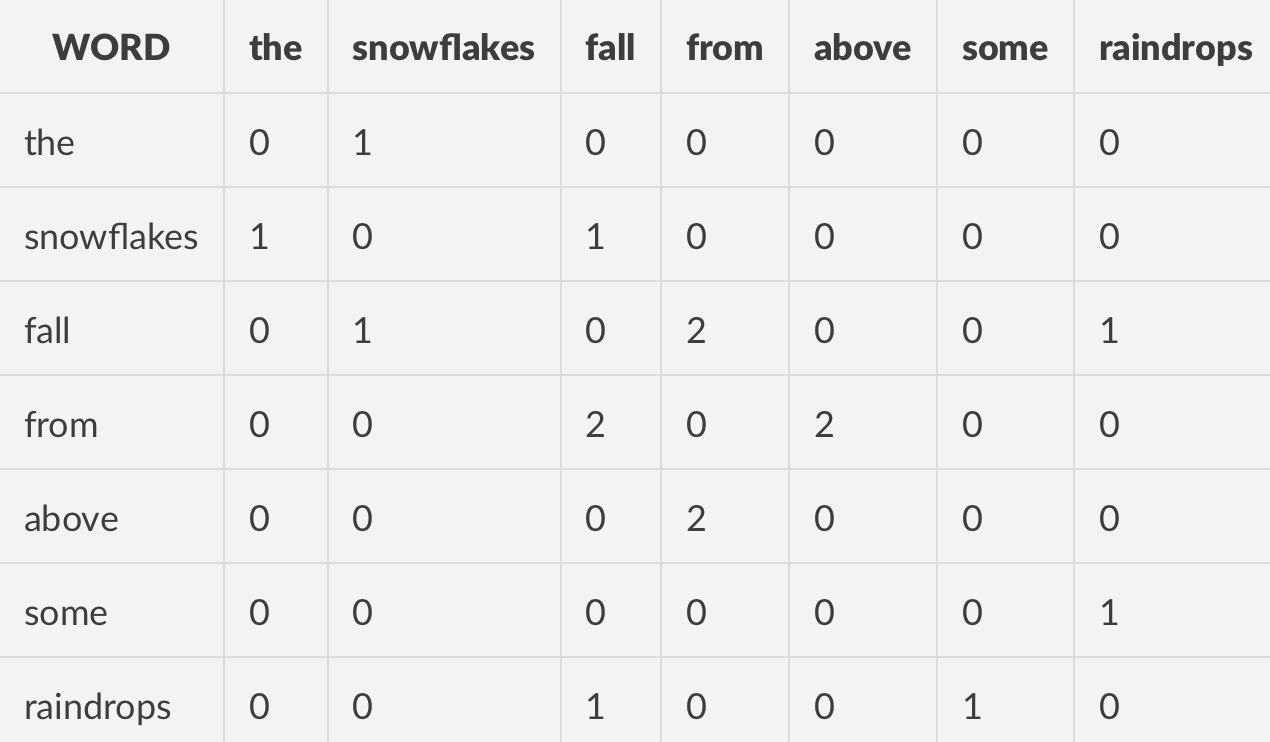# Word Vectors Simplified

Keywords: #nlp #word-vectors

Note: This blog post is based on assignment 1 from the Stanford CS 224N NLP And Deep Learning Course which is available here. I highly recommend this course for people beginning NLP as it is freely available, has great content and is great fun to follow!

# Introduction

Below I hope to outline the concept of word vectors and to show how useful they can be. With the aim of keeping this concise, I will not be discussing implementation details. However these can be found in the course linked at the beginning of this post.

Word vectors (or word embeddings) are vector representations of the meaning of a given word.

# Finding Word Meaning From Surrounding Words

Word vectors commonly rely on the fact that words which are commonly found next or close to other words are usually linguistically related (either syntactically or semantically).

For example, it is natural to think that you would find the word “trombone” in contexts with a musical theme so you could predict other words which might be in the text such as “instrument” or “song”. You would probably also say that it is unlikely that you would find the word “kebab” around the word “trombone” because of their very different meanings.

In real NLP practices, many of the words surrounding a word are used to create a mathematical definition of the word. This number of words is commonly referred to as the window.

Here is an example of an active window when our centre word is “snowflakes” and with a window size of 1:# Co-Occurrence Matrices

An easy way to represent how frequently words are in the context of other words is to use a co-occurrence matrix. The simple way to think of a co-occurrence matrix is that it is a table where the values are counters of how many times two words co-occur!

1. “the snowflakes fall from above”
2. “some raindrops fall from above”

Given the sentences above with a window size 1, we would get the following co-occurrence matrix:# Synonyms from Vector Similarity

Once you have the vector representations of each word calculated, you effectively have vector definitions for each word. It turns out that you can use vector calculations to interact with these representations. For example, many people learn how to find angles between vectors using cosine similarity.

$$similarity = cos(\theta) = \frac{\mathbf{A \cdot B}}{\Vert A \Vert \Vert B \Vert}$$

The more similar the two vectors, the more closely related the two words are likely to be in meaning.

# Using Vector Maths to Find Semantic Relations

An interesting find which is discussed in the course practical this is based on, revolves around the difference in vectors and using vector representations to explore relations between words as well as meanings.

One of the examples uses the the roles of King and Queen and gensim’s most_similar() to investigate. They show that the difference between man and king should be the same vector difference between queen and woman. Hence:

$$woman + king - man = queen$$

# Flattening Matrices for Graphical Representation

Once we have a co-occurrence matrix we can see that the dimensions are too large to display on a standard 2D or 3D graph (and would but much much larger in a practical situation with a corpus consisting of more than 7 words)!

In order to represent our matrix on a graph we will have to shrink the matrix so that we have appropriate x and y coordinates.

A popular way to do this is to use SVD (singular value decomposition) .

Since this post is about keeping it simple I won’t go into how SVD works but here is a useful resource from MIT on understanding SVD if you want to delve deeper. The key thing for us is that SVD will help us through dimensionality reduction (going from an n x n matrix to a 2 x n matrix).